# Isomni'hack 2017 teaser mindreader writeup

Machines infected lots of Android smartphones and try to collect information on human behaviour...

Have a look to their application and try to steal information on them.

So we have an android application file. Let's decompile its code!

First, we need to translate Dalvik bytecode to equivalent Java bytecode. I used enjarify for this:

``````➜ git clone https://github.com/google/enjarify
➜ cd enjarify
``````

And now we can decompile java bytecode by using jd-gui. Let's see what we have.

The first intresting function is `readMind`:

``````static String device = "000000000000000";
...
{
localObject1 = device;
String str1 = jsonify((String)localObject1); // encode to json {"device": "..."}
byte[] arrayOfByte1 = str1.getBytes();
byte[] arrayOfByte2 = new byte[arrayOfByte1.length];
localObject1 = getApplicationContext();
encrypt((Context)localObject1, arrayOfByte1, arrayOfByte2);
int i = 0;
localObject1 = null;
String str2 = Base64.encodeToString(arrayOfByte2, 0);
... // Send HTTP-request with str2 as parameter to server
}
``````

Here we can see that string with json `{"device": "000000000000000"}` is encrypted, encoded to base64 and then sent to the server. And `encrypt` function looks like this:

``````public native int encrypt(Context paramContext, byte[] paramArrayOfByte1, byte[] paramArrayOfByte2);
``````

And above this we have lines:

``````static
{
}
``````

So as we can see `encrypt` function is implemented in library `libnative-lib.so`. Let's find it.

First, we should extract application files. I used apktool for this:

``````➜ apktool d mindreader-c3df7f2c966238cc8f4d4327dc1dca8b8b5a69d702f966963c828c965ebbf516.apk
➜ file libnative-lib.so
libnative-lib.so: ELF 32-bit LSB shared object, ARM, EABI5 version 1 (SYSV), dynamically linked, interpreter /system/bin/linker, BuildID[sha1]=f092f48095eec3cb0c6dd8eddec9994c2b3e01b4, stripped
``````

Now we should find `encrypt` function in this library. As `encrypt` is called from java code it seems that it should use JNI (Java Native Interface). So, according to Oracle documentation name of `encrypt` function in the library will be like `Java_ch_scrt_hiddenservice_MainActivity_encrypt` (`ch.scrt.hiddenservice` - name of the application package, `MainActivity` - name of class).

In Ida Pro this function looks like this:

``````int __fastcall Java_ch_scrt_hiddenservice_MainActivity_encrypt(int a1, int a2, int a3, int a4, int a5)
{
...

v14 = a4;
v5 = a3;
v6 = a1;
v13 = a1;
v7 = 0;
v18 = 0;
v12 = (*(int (**)(void))(*(_DWORD *)a1 + 684))();
v17 = (*(int (__fastcall **)(int, int, char *))(*(_DWORD *)v6 + 736))(v6, v14, &v18);
v16 = (*(int (__fastcall **)(int))(*(_DWORD *)v6 + 736))(v6);
sub_4A68();
v8 = sub_4AC4(v6, v5);
v19 = v8;
v20 = v8 >> 16;
v21 = HIBYTE(v8);
if ( v12 > 0 )
{
v15 = dword_1D0F8;
do
{
v9 = *(_BYTE *)(v17 + v7);
j_j_j___aeabi_idivmod(v7, 80);
*(_BYTE *)(v16 + v7) = *((_BYTE *)&v19 + v7 % 4) ^ *(_BYTE *)(v15 + v10) ^ v9;
++v7;
}
while ( v12 != v7 );
}
(*(void (__fastcall **)(int, int, int, _DWORD))(*(_DWORD *)v13 + 768))(v13, v14, v17, 0);
(*(void (__fastcall **)(int, int, int, _DWORD))(*(_DWORD *)v13 + 768))(v13, a5, v16, 0);
if ( _stack_chk_guard != v22 )
j_j___stack_chk_fail();
return 0;
}
``````

Also according to JNI Oracle documentation the first argument of this function is `JNIEnv* env` and the second is `jobject obj`. The rest of arguments is arguments from java i.e. `Context paramContext, byte[] paramArrayOfByte1, byte[] paramArrayOfByte2)`. Now our function looks like this:

``````int __fastcall Java_ch_scrt_hiddenservice_MainActivity_encrypt(int env, int obj, int paramContext, int paramArrayOfByte1, int paramArrayOfByte2)
{
...

paramArrayOfByte1_1 = paramArrayOfByte1;
paramContext_1 = paramContext;
env_1 = env;
env_2 = env;
v7 = 0;
v18 = 0;
v12 = (*(int (**)(void))(*(_DWORD *)env + 684))();
v17 = (*(int (__fastcall **)(int, int, char *))(*(_DWORD *)env_1 + 736))(env_1, paramArrayOfByte1_1, &v18);
v16 = (*(int (__fastcall **)(int))(*(_DWORD *)env_1 + 736))(env_1);
sub_4A68();
v8 = sub_4AC4(env_1, paramContext_1);
v19 = v8;
v20 = v8 >> 16;
v21 = HIBYTE(v8);
if ( v12 > 0 )
{
v15 = dword_1D0F8;
do
{
v9 = *(_BYTE *)(v17 + v7);
j_j_j___aeabi_idivmod(v7, 80);
*(_BYTE *)(v16 + v7) = *((_BYTE *)&v19 + v7 % 4) ^ *(_BYTE *)(v15 + v10) ^ v9;
++v7;
}
while ( v12 != v7 );
}
(*(void (__fastcall **)(int, int, int, _DWORD))(*(_DWORD *)env_2 + 768))(env_2, paramArrayOfByte1_1, v17, 0);
(*(void (__fastcall **)(int, int, int, _DWORD))(*(_DWORD *)env_2 + 768))(env_2, paramArrayOfByte2, v16, 0);
if ( _stack_chk_guard != v22 )
j_j___stack_chk_fail();
return 0;
}
``````

Better but still not readable because of many function calls like `(*(int (__fastcall **)(int, int, char *))(*(_DWORD *)env_1 + 736))` i.e. by offset in struct `JNIEnv *env`. We need to find function names by their offsets in struct `JNIEnv`. All JNI functions are listed here. But I found cool Ida script IDA_JNI_Rename on GitHub that helps to do renamamings. After using it our function will look like this:

``````int __fastcall Java_ch_scrt_hiddenservice_MainActivity_encrypt(int env, int obj, int paramContext, int paramArrayOfByte1, int paramArrayOfByte2)
{
...
paramArrayOfByte1_1 = paramArrayOfByte1;
paramContext_1 = paramContext;
env_1 = env;
env_2 = env;
v7 = 0;
v18 = 0;
v12 = (*(int (**)(void))(*(_DWORD *)env + jni_GetArrayLength))();
v17 = (*(int (__fastcall **)(int, int, char *))(*(_DWORD *)env_1 + jni_GetByteArrayElements))(
env_1,
paramArrayOfByte1_1,
&v18);
v16 = (*(int (__fastcall **)(int))(*(_DWORD *)env_1 + jni_GetByteArrayElements))(env_1);
sub_4A68();
v8 = sub_4AC4(env_1, paramContext_1);
v19 = v8;
v20 = v8 >> 16;
v21 = HIBYTE(v8);
if ( v12 > 0 )
{
v15 = dword_1D0F8;
do
{
v9 = *(_BYTE *)(v17 + v7);
j_j_j___aeabi_idivmod(v7, 80);
*(_BYTE *)(v16 + v7) = *((_BYTE *)&v19 + v7 % 4) ^ *(_BYTE *)(v15 + v10) ^ v9;
++v7;
}
while ( v12 != v7 );
}
(*(void (__fastcall **)(int, int, int, _DWORD))(*(_DWORD *)env_2 + jni_ReleaseByteArrayElements))(
env_2,
paramArrayOfByte1_1,
v17,
0);
(*(void (__fastcall **)(int, int, int, _DWORD))(*(_DWORD *)env_2 + jni_ReleaseByteArrayElements))(
env_2,
paramArrayOfByte2,
v16,
0);
if ( _stack_chk_guard != v22 )
j_j___stack_chk_fail();
return 0;
}
``````

Now we can assume that `paramArrayOfByte1` is `plaintext` and `paramArrayOfByte2` is `ciphertext`. Let's do some more renamings:

``````int __fastcall Java_ch_scrt_hiddenservice_MainActivity_encrypt(int env, int obj, int paramContext, int plaintext, int ciphertext)
{
...
paramArrayOfByte1_1 = plaintext;
paramContext_1 = paramContext;
env_1 = env;
env_2 = env;
i = 0;
v18 = 0;
plaintext_len = (*(int (**)(void))(*(_DWORD *)env + jni_GetArrayLength))();
plaintext_bytes = (*(int (__fastcall **)(int, int, char *))(*(_DWORD *)env_1 + jni_GetByteArrayElements))(
env_1,
paramArrayOfByte1_1,
&v18);
ciphertext_bytes = (*(int (__fastcall **)(int))(*(_DWORD *)env_1 + jni_GetByteArrayElements))(env_1);
sub_4A68();
some_int = sub_4AC4(env_1, paramContext_1);
some_int_1 = some_int;
v20 = some_int >> 16;
v21 = HIBYTE(some_int);
if ( plaintext_len > 0 )
{
v15 = dword_1D0F8;
do
{
v9 = *(_BYTE *)(plaintext_bytes + i);
j_j_j___aeabi_idivmod(i, 80);
*(_BYTE *)(ciphertext_bytes + i) = *((_BYTE *)&some_int_1 + i % 4) ^ *(_BYTE *)(v15 + v10) ^ v9;
++i;
}
while ( plaintext_len != i );
}
(*(void (__fastcall **)(int, int, int, _DWORD))(*(_DWORD *)env_2 + jni_ReleaseByteArrayElements))(
env_2,
paramArrayOfByte1_1,
plaintext_bytes,
0);
(*(void (__fastcall **)(int, int, int, _DWORD))(*(_DWORD *)env_2 + jni_ReleaseByteArrayElements))(
env_2,
ciphertext,
ciphertext_bytes,
0);
if ( _stack_chk_guard != v22 )
j_j___stack_chk_fail(_stack_chk_guard - v22);
return 0;
}
``````

So, the encryption algoritm looks like this:

``````int some_int = sub_4AC4(env_1, paramContext_1);
int dword_1D0F8 = ?;
for (i = 0; i < plaintext_len; i++) {
ciphertext[i] = plaintext[i] ^ some_int[i % 4] ^ dword_1D0F8[i % 80];
}
``````

Cool, but we don't have values `some_int` and `dword_1D0F8`. At this point I decided that it would be easier to place a breakpoint here and just copy this values from memory because I'm lazy :) To do this I used android emulator `armeabi-v7a`: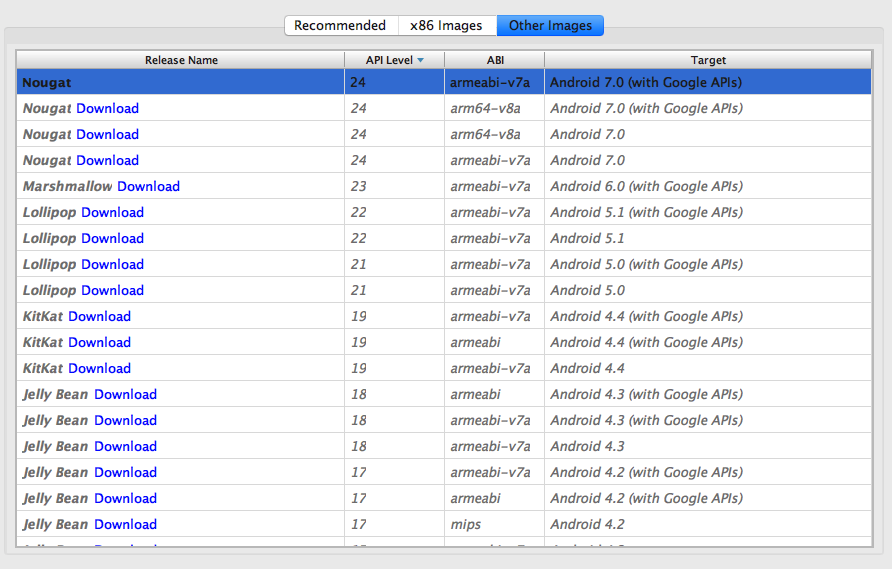Start emulator with the command:

``````➜ emulator -avd Nexus_5_API_24
``````

Then install application to emulator by drag'n'dropping APK-file to emulator's window.

After that set up Ida Dalvik debugger as described here and place a breakpoint on `encrypt` in `readMind` function: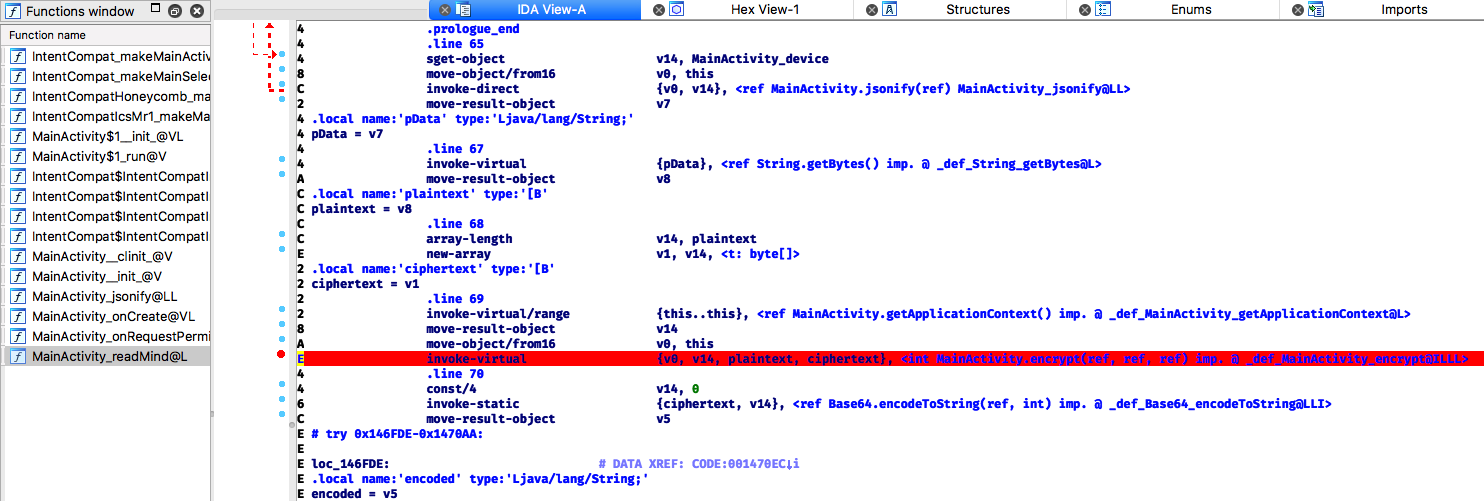Then open another Ida instance with `libnative-lib.so`, set up remote android debugger as described here and place a breakpoint before encryption starts: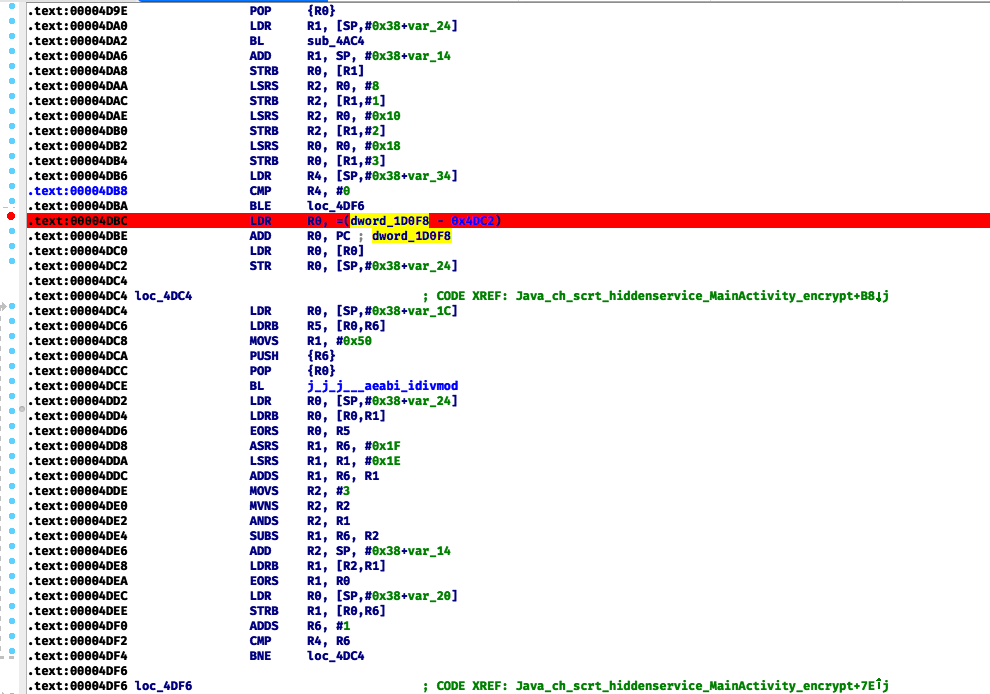After that start Ida with Dalvik debugger and wait until program stops and then start remote android debugger and attach to application process: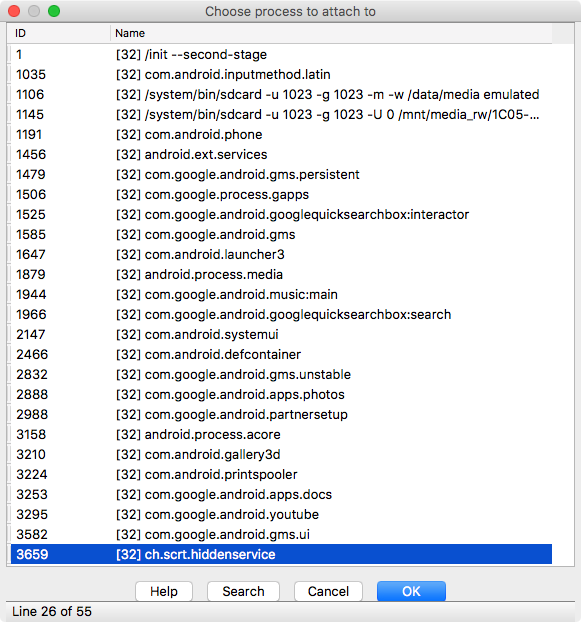Next, press continue (F9) in the first Ida instance (Dalvik debugger) and wait until breakpoint fires in the second instance.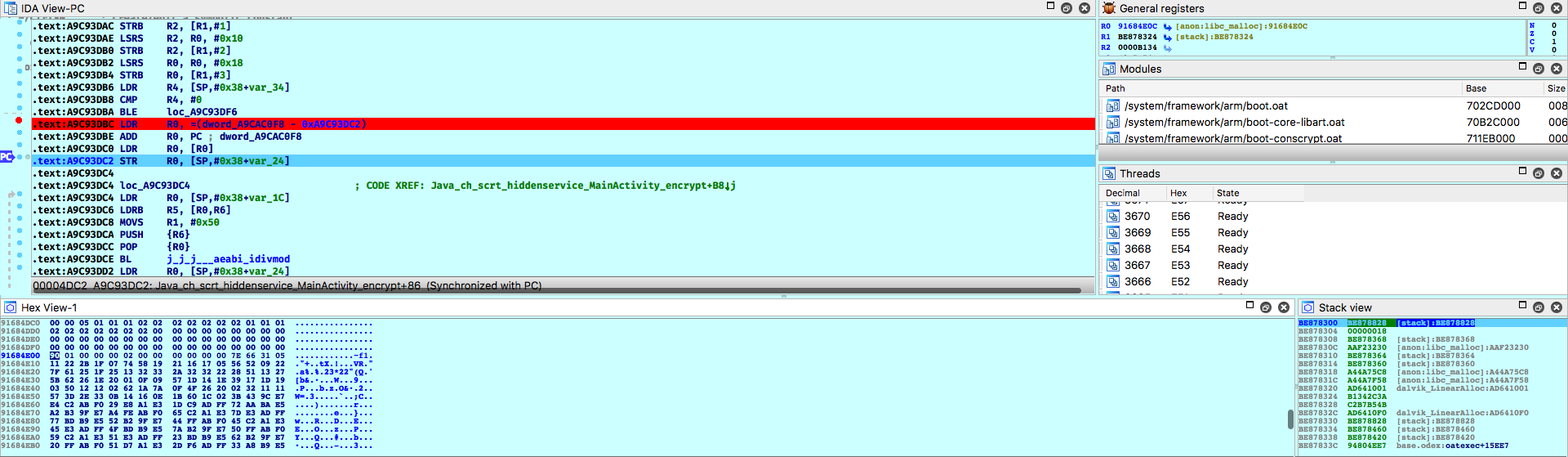Ok, now we can just copy values of `some_int` and `dword_1D0F8`.

`dword_1D0F8` (started with `7E 66 31 05`):and `some_int = 0xb1342c3a`:So we can rewrite encrypion in python:

``````import json
import base64

table = [
0x7e, 0x66, 0x31, 0x05, 0x11, 0x22, 0x2b, 0x1f,
0x07, 0x74, 0x58, 0x19, 0x21, 0x16, 0x17, 0x05,
0x56, 0x52, 0x09, 0x22, 0x7f, 0x61, 0x25, 0x1f,
0x25, 0x13, 0x32, 0x33, 0x2a, 0x32, 0x32, 0x22,
0x28, 0x51, 0x13, 0x27, 0x5b, 0x62, 0x26, 0x1e,
0x20, 0x01, 0x0f, 0x09, 0x57, 0x1d, 0x14, 0x1e,
0x39, 0x17, 0x1d, 0x19, 0x03, 0x50, 0x12, 0x12,
0x02, 0x62, 0x1a, 0x7a, 0x0f, 0x4f, 0x26, 0x20,
0x02, 0x32, 0x11, 0x11, 0x57, 0x3d, 0x2e, 0x33,
0x0b, 0x14, 0x16, 0x0e, 0x1b, 0x60, 0x1c, 0x02,
]

crc = [ 0x3a, 0x2c, 0x34, 0xb1 ]

def encrypt(p):
c =  * len(p)
for i in range(len(p)):
c[i] = chr(ord(p[i]) ^ crc[i % 4] ^ table[i % len(table)])
return "".join(c)

def encode(data):
return base64.b64encode(encrypt(json.dumps(data)))
``````

To check it we can intercept HTTP-request from emulator and get:

``````GET /?a=1&c=P2hh0V1nfMsfYk6YKwoThFxODaN1fSGeLw8k%2Fw%3D%3D%0A HTTP/1.1
User-Agent: Dalvik/2.1.0 (Linux; U; Android 7.0; sdk_google_phone_armv7 Build/NYC)
Connection: close
``````

So, we can check correctness of python script like this:

``````test_in = '{"device":"000000000000000"}'
test_out = base64.b64decode("P2hh0V1nfMsfYk6YKwoThFxODaN1fSGeLw8k/w==")
assert(encrypt(test_in) == test_out)
``````

Now we can try all requests from application:

``````URL = "http://mindreader.teaser.insomnihack.ch"

data = {
"device": device_id
}
params = {
"a": 1,
"c": encode(data)
}
r = requests.get(URL, params=params)
return r

def sms_send(device_id, date, sender, body):
data = {
"device": device_id,
"date": 0,
"sender": sender,
"body": body
}
params = {
"a": 2,
"c": encode(data)
}
r = requests.get(URL, params=params)
return r
``````

`sms_send` request I found in file `SMSReceiver.java` in JD-GUI.

After playing a little bit with this two requests I found that parameter `sender` in `sms_send` is vulnerable to SQL injection (time-based). So, after gettting all nessesary table names and column names I got a flag:

``````➜ python solve.py